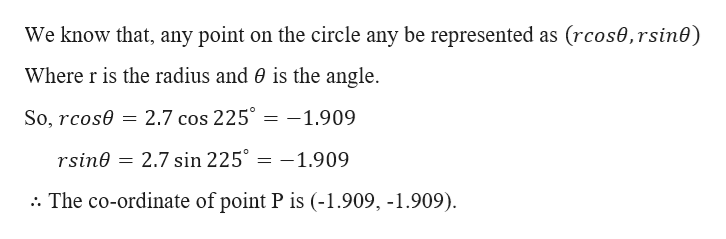# Find the coordinates of the point P at an angle of 225 degrees on a circle of radius 2.7. Round your answers to the three decimal places. Enter a point as (a, b).

Question
17 views

Find the coordinates of the point P at an angle of 225 degrees on a circle of radius 2.7. Round your answers to the three decimal places. Enter a point as (a, b).

check_circle

Step 1

Given,

The poi...help_outlineImage TranscriptioncloseWe know that, any point on the circle any be represented as (rcos0, rsin0) Where r is the radius and 0 is the angle 2.7 cos 225° So, rcose -1.909 2.7 sin 225° rsine -1.909 :. The co-ordinate of point P is (-1.909, -1.909) fullscreen

### Want to see the full answer?

See Solution

#### Want to see this answer and more?

Solutions are written by subject experts who are available 24/7. Questions are typically answered within 1 hour.*

See Solution
*Response times may vary by subject and question.
Tagged in

### Trigonometric Ratios